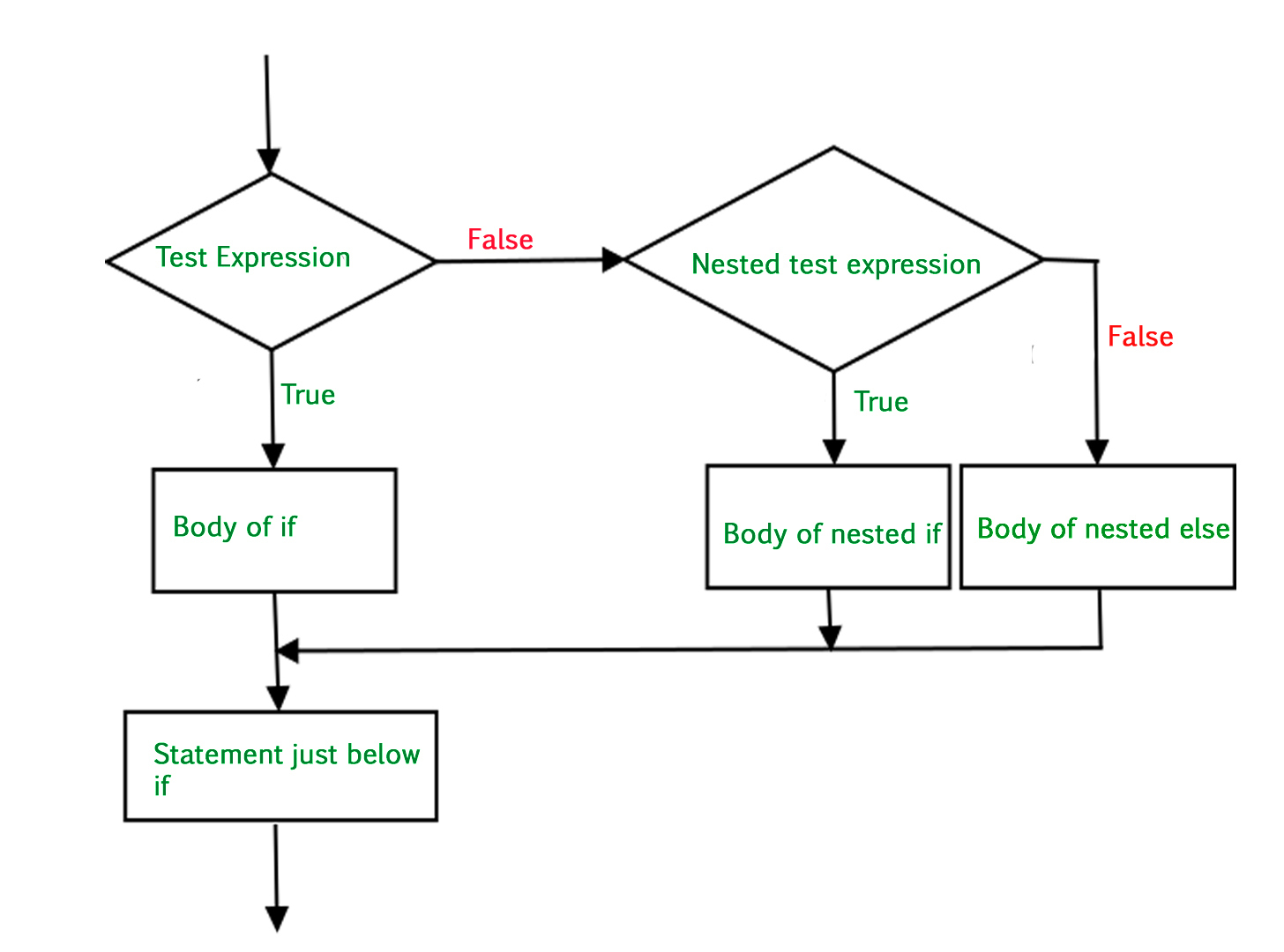# Nested-if statement in Python

• Last Updated : 26 Mar, 2020

There come situations in real life when we need to make some decisions and based on these decisions, we decide what should we do next. Similar situations arise in programming also where we need to make some decisions and based on these decisions we will execute the next block of code. This is done with the help of decision-making statements in Python.

Example:

Attention geek! Strengthen your foundations with the Python Programming Foundation Course and learn the basics.

To begin with, your interview preparations Enhance your Data Structures concepts with the Python DS Course. And to begin with your Machine Learning Journey, join the Machine Learning - Basic Level Course

 `# Python program to demonstrate``# decision making`` ` `i ``=` `20``; ``if` `(i < ``15``): ``    ``print` `(``"i is smaller than 15"``) ``    ``print` `(``"i'm in if Block"``) ``else``: ``    ``print` `(``"i is greater than 15"``) ``    ``print` `(``"i'm in else Block"``) ``print` `(``"i'm not in if and not in else Block"``) `

Output:

```i is greater than 15
i'm in else Block
i'm not in if and not in else Block```

## Nested-if Statement

We can have an if…elif…else statement inside another if…elif…else statement. This is called nesting in computer programming. Any number of these statements can be nested inside one another. Indentation is the only way to figure out the level of nesting. This can get confusing, so it must be avoided if we can.

Syntax:

```if (condition1):
# Executes when condition1 is true
if (condition2):
# Executes when condition2 is true
# if Block is end here
# if Block is end here```

FlowChartExample 1:

 `# Python program to demonstrate``# nested if statement`` ` `  ` `num ``=` `15``if` `num >``=` `0``:``    ``if` `num ``=``=` `0``:``        ``print``(``"Zero"``)``    ``else``:``        ``print``(``"Positive number"``)``else``:``    ``print``(``"Negative number"``)`

Output:

```Positive number
```

Example 2:

 `# Python program to demonstrate``# nested if statement`` ` ` ` `i ``=` `13`` ` `if` `(i ``=``=` `13``): ``    ``#  First if statement ``    ``if` `(i < ``15``): ``        ``print` `(``"i is smaller than 15"``) ``         ` `    ``# Nested - if statement ``    ``# Will only be executed if statement above ``    ``# it is true ``    ``if` `(i < ``12``): ``        ``print` `(``"i is smaller than 12 too"``) ``    ``else``: ``        ``print` `(``"i is greater than 12 and smaller than 15"``)`

Output:

```i is smaller than 15
i is greater than 12 and smaller than 15
```

My Personal Notes arrow_drop_up